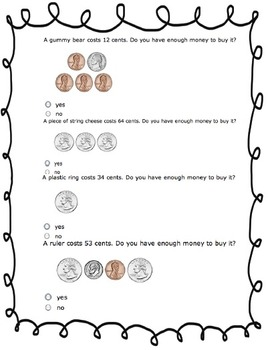Math Practice or Homework WorksheetsSubject
Resource Type
Product Rating
4.0
2 Ratings
File Type

PDF (Acrobat) Document File

Be sure that you have an application to open this file type before downloading and/or purchasing.

413 KB|4 pages
Share
Product Description
CCSS.Math.Content.1.OA.A.1 Use addition and subtraction within 20 to solve word problems involving situations of adding to, taking from, putting together, taking apart, and comparing, with unknowns in all positions, e.g., by using objects, drawings, and equations with a symbol for the unknown number to represent the problem.1

Understanding tens and ones
CCSS.Math.Content.1.NBT.B.2 Understand that the two digits of a two-digit number represent amounts of tens and ones.

Counting Coins
CCSS.Math.Content.1.OA.C.6 Add and subtract within 20, demonstrating fluency for addition and subtraction within 10. Use strategies such as counting on; making ten (e.g., 8 + 6 = 8 + 2 + 4 = 10 + 4 = 14); decomposing a number leading to a ten (e.g., 13 – 4 = 13 – 3 – 1 = 10 – 1 = 9); using the relationship between addition and subtraction (e.g., knowing that 8 + 4 = 12, one knows 12 – 8 = 4); and creating equivalent but easier or known sums (e.g., adding 6 + 7 by creating the known equivalent 6 + 6 + 1 = 12 + 1 = 13).

Odd and Even
CCSS.Math.Content.2.OA.C.3 Determine whether a group of objects (up to 20) has an odd or even number of members, e.g., by pairing objects or counting them by 2s; write an equation to express an even number as a sum of two equal addends.
***^ This is a second grade standard!
Total Pages
4 pages
N/A
Teaching Duration
N/A
Report this Resource to TpT
Reported resources will be reviewed by our team. Report this resource to let us know if this resource violates TpT’s content guidelines.